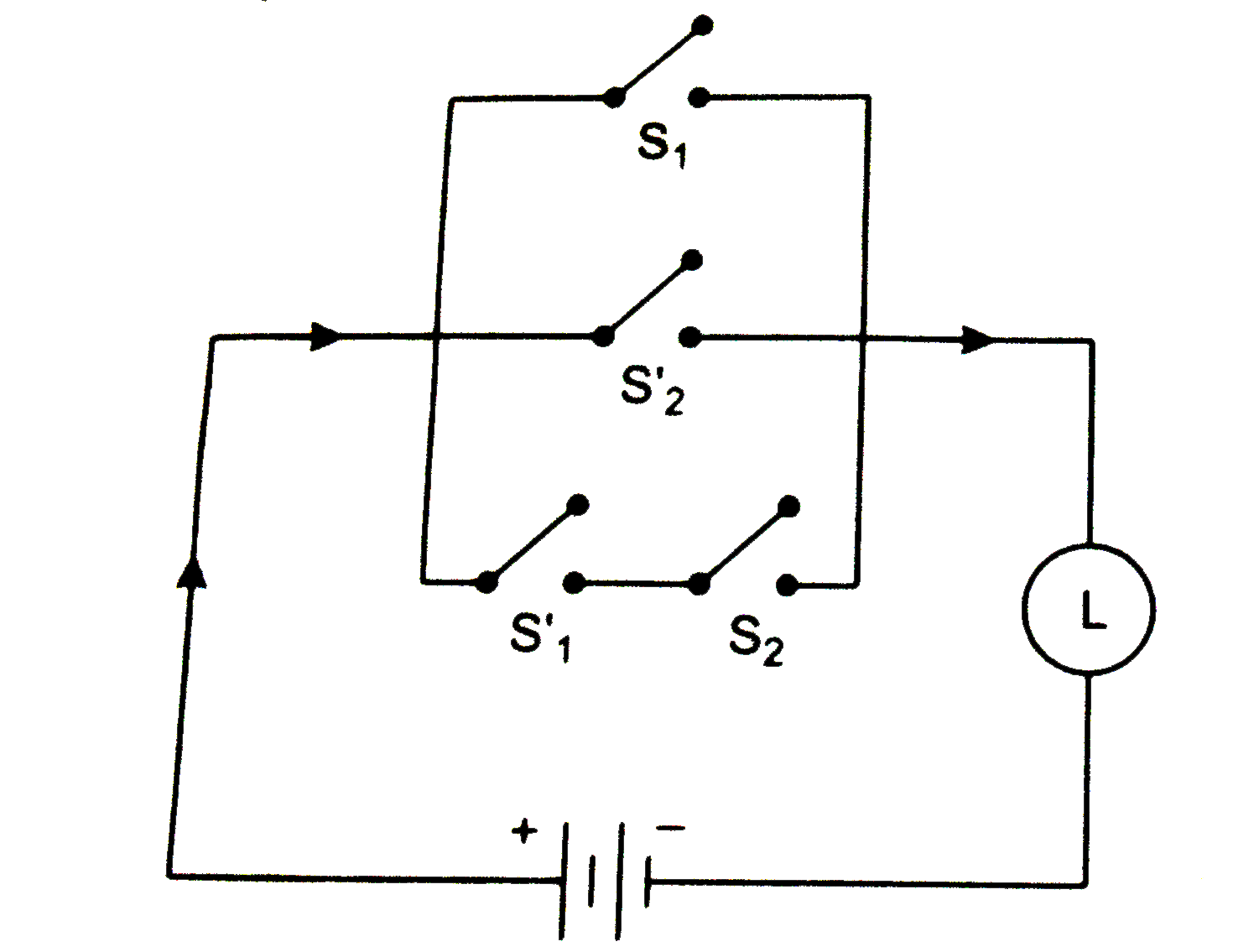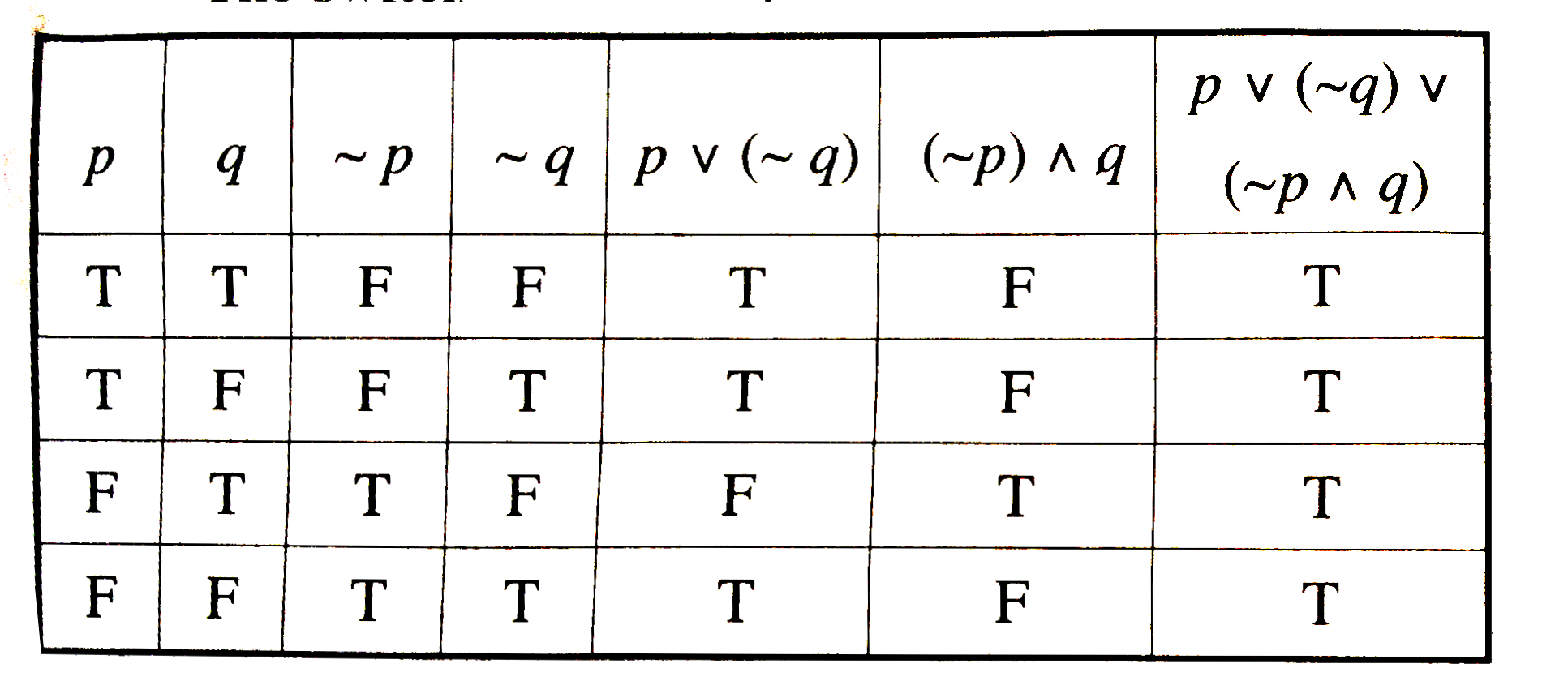# Express the following switching circuit in symbolic form of logic . Costruct its switching table and write your conlusion form it :

1.1k views

closed
Express the following switching circuit in symbolic form of logic . Costruct its switching table and write your conlusion form it :by (91.7k points)
selected

Let p : the switch S_(1) is closed.
q : the switch S_(2) is closed.
 ~p: the switch S_(1) is closed or the switch S_(1) is open.
~q : the switch S_(2) is closed or the switch S_(2) is open.
From the given circuit the lamp is on if (S_(1) is closed) or (S_(2) is closed ) or (S_(1) is closed and S_(2) is closed (.
Hence , the symbolic form of the given circuit is p vv(~q)vv(~p ^^ q)
The switching table is :This circuit represents a tautolgy.
Hence , the given circuit is always closed (i.e., on)